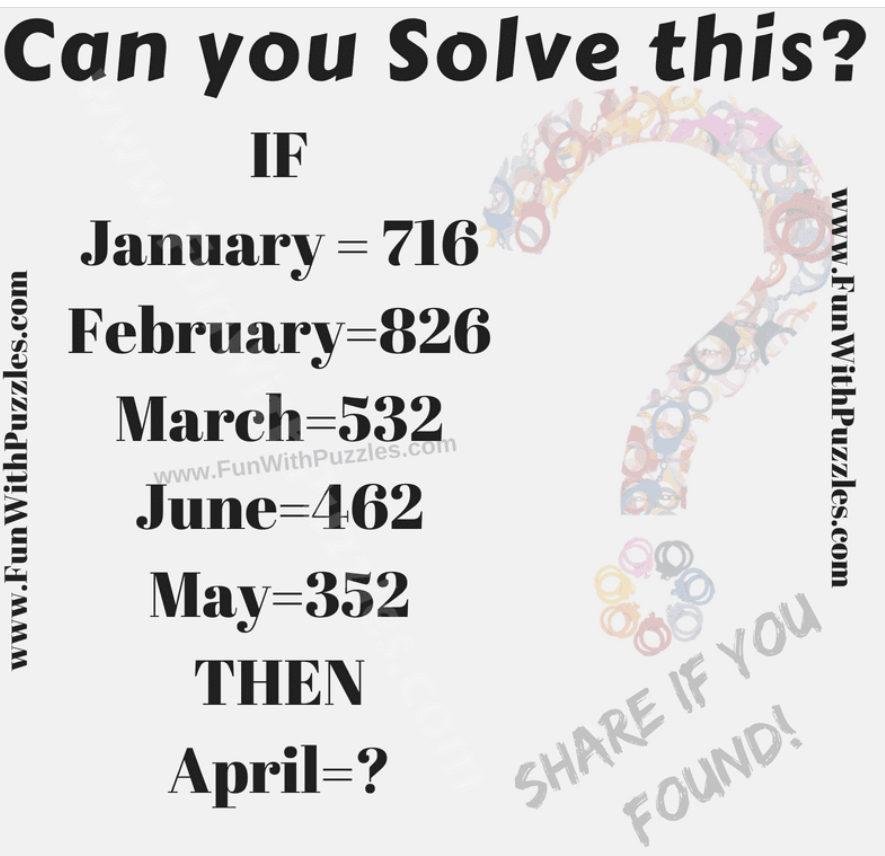# REAL IQ TEST HOW SMART ARE YOU???There is no better way to test your IQ than to solve a puzzle and see how well you do. There is a puzzle that is taking the Internet by storm and leaving those who cannot solve it perplexed.

There are many puzzles that are difficult to solve, but this one seems almost impossible. Is this puzzle really as difficult as everyone said? If you still cannot solve it, you can also find the answer and the process on the Internet.In this Brain Teaser there are equations which contains month name and number. There is Logical or Mathematical relationship between both sides of the equation. Can you find this relationship and solve this IQ Test Brain Teaser?

IF January=716, February=826, March=532, June=462 Then April=? Can you solve this IQ Test Brain Teaser?The Answer is 541. First Digit is the number of letters in the word on left side of equation Second digit is sequence of the month in the calendar Third Digit is the absolute difference between first two digits